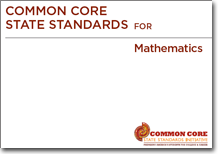# About Common Core State Standards"These Standards define what students should understand and be able to do in their study of mathematics. Asking a student to understand something means asking a teacher to assess whether the student has understood it. But what does mathematical understanding look like? One hallmark of mathematical understanding is the ability to justify, in a way appropriate to the student's mathematical maturity, why a particular mathematical statement is true or where a mathematical rule comes from. There is a world of difference between a student who can summon a mnemonic device to expand a product such as (a + b)(x + y) and a student who can explain where the mnemonic comes from. The student who can explain the rule understands the mathematics, and may have a better chance to succeed at a less familiar task such as expanding (a + b + c)(x + y). Mathematical understanding and procedural skill are equally important, and both are assessable using mathematical tasks of sufficient richness."